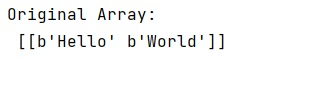# Weird behaviour initializing a NumPy array of string data

Here, we will understand the working of initializing a NumPy array of string data in Python.
Submitted by Pranit Sharma, on February 20, 2023

NumPy is an abbreviated form of Numerical Python. It is used for different types of scientific operations in python. Numpy is a vast library in python which is used for almost every kind of scientific or mathematical operation. It is itself an array which is a collection of various methods and functions for processing the arrays.

## Initializing a NumPy array of string data

Many times, programmers face some trivial trouble with numpy when the array contains string data.

For example, if we create an empty array of data type 'str' and then if we assign some string as the elements in the array, it will not result in a NumPy array with string elements.

This is because numpy requires string arrays to have a fixed maximum length. When we create an empty array with dtype=str, it sets this maximum length to 1 by default. We can see if we do arr.dtype; it will show "|S1", which represents a "one-character string". Hence, we can pass an explicit datatype with your maximum length by giving a required length.

Let us understand with the help of an example,

## Python code to demonstrate the weird behaviour initializing a NumPy array of string data

```# Import numpy
import numpy as np

# Creating a numpy array of strings
arr = np.empty([1, 2], dtype="S10")

# Assigning elements
arr[0,0] = 'Hello'
arr[0,1] = 'World'

# Display original array
print("Original Array:\n",arr)
```

Output: